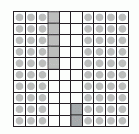Name: ___________________Date:___________________

 Email us to get an instant 20% discount on highly effective K-12 Math & English kwizNET Programs!

### Grade 5 - Mathematics2.14 Decimal Sums

 Write the decimals one below the other. Note: The point must be aligned and the numbers one below the other. Example: Add 0.35 and 0.42 Consider a square being divided into 100 equal parts.Shade 3 tenths and colour five hundreths which shows 0.35. Shade 4 tenths and colour 2 hundreths which shows 0.42. Count the total number of tenths and hundreths in the square.Answer: 0.77 Directions: Add the following decimal numbers. Also write at least ten examples of your own.
 Question 1: 7134.9 + 2272.8

 Question 2: 8.1957 + 7.2515

 Question 3: 533.12 + 518.24

 Question 4: 82.000 + 95.057

 Question 5: 2497.2 + 6926.3

Question 6: This question is available to subscribers only!

Question 7: This question is available to subscribers only!

Question 8: This question is available to subscribers only!

Question 9: This question is available to subscribers only!

Question 10: This question is available to subscribers only!